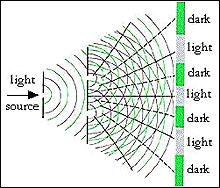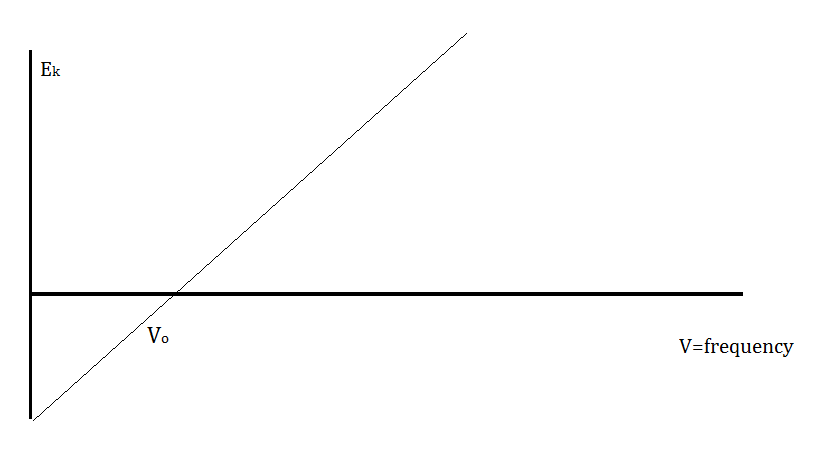# 12.2: The Nature of Matter

In 1923, Louis de Broglie, a French physicist, proposed a hypothesis to explain the theory of the atomic structure.By using a series of substitution de Broglie hypothesizes particles to hold properties of waves. Within a few years, de Broglie's hypothesis was tested by scientists shooting electrons and rays of lights through slits. What scientists discovered was the electron stream acted the same was as light proving de Broglie correct.

## Definition of Wave-Particle Duality

The behaviors of the electron does not allow for it to be observable as a particle and as a wave. The two sided nature of the electron is known as the Wave-Particle Duality: The property of particles behaving as waves and the property of waves behaving as particles as well as waves. Although the duality is not very effective in large matter. The wave characteristic of the electron implicates many of the electron's particle behaviors.

Planck's Hypothesis of the Quantum Theory states that energy is emitted in quanta, little packets of energy, instead of a continuous emission. He stated that energy emitted is related to the frequency of the light emitted. Planck's hypothesis states that a quantum of energy was related to the frequency by his equation $$E = h\nu$$.

## Waves & Particles Behaviors of Light

An easy way to prove the duality between a particle and a wave is to observe light. At the time, many scientists believed that light is a wave. Since light is like waves, it has the ability to diffract, reflect, refract, and interfere etc . . . Yet, light behaved strangely at certain times, and scientists were befuddled until . . .Diffraction Interference

Albert Einstein's theory of photoelectric effect contributed greatly to De Broglie's Theory and was a proof that waves and particles could overlap. Light can also be observed as a particle known as photon. When light is shown on certain objects, the electrons will be released. Certain amounts of energy is needed to remove an electron from the surface of a substance. So, if a photon of greater energy than that of an electron hits a solid that electron will be emitted.The following picture also describes threshold Vo, where one photon did not have enough intensity to throw off an electron.

When the electrons are release, they also release kinetic energy. Classical wave theory states the greater the intensity the greater the energy. Since energy of a wave is directly proportional to its amplitude, it was puzzling for scientists to find brighter lights(higher intensity) did not affect its overall kinetic energy.

However, scientists did discover that frequency of light effectively changed the amount of kinetic energy. Since certain objects do not emit electrons under certain frequencies, a threshold, V0, is used. This threshold was used to describe the amount of kinetic energy needed for a photon to throw off an electron. They arrived at a linear relation for frequency and kinetic energy given by the rough sketch ofThe slope of this line was confirm to be Planck's Constant, h = 6.63 x 10-34

Using the graph, we are given the same equation as before: Ek = hv. Since the energy of waves and energy of light do not coincide, we can rule that light is a particle that contains the property of waves.

## De Broglie Wavelength

De Broglie derived his equation using well established theories through the following series of substitutions:

1. De Broglie first used Einstein's famous equation relating matter and energy:

$E = mc^2$

E= energy, m = mass, c = speed of light

2. Using Planck's theory which states every quantum of a wave has a discrete amount of energy given by Planck's equation:

$E= h \nu$

E = energy, h = Plank's constant(6.62607 x 10-34 J s), υ = frequency

3. Since de Broglie believes particles and wave have the same traits, the two energies would be the same:

$mc^2 = h\nu$

4. Because real particles do not travel at the speed of light, De Broglie subsituted v, velocity, for c, the speed of light.

$mv^2 = h\nu$

5. Through the equation $$\lambda$$, de Broglie substituted $$v/\lambda$$ for $$\nu$$ and arrived at the final expression that relates wavelength and particle with speed.

$mv^2 = \dfrac{hv}{\lambda}$

Hence:

$\lambda = \dfrac{hv}{mv^2} = \dfrac{h}{mv}$

Although De Broglie was credited for his hypothesis, he had no actual experimental evidence for his conjecture. In 1927, Clinton J. Davisson and Lester H. Germer shot electron particles onto onto a nickel crystal. What they see is the diffraction of the electron similar to waves diffractions against crystals(x-rays). In the same year, an English physicist, George P. Thomson fired electrons towards thin metal foil providing him with the same results as Davisson and Germer.

## Quantum Theory Made Easy Video: Dr. Quantum

• De Broglie's Theory can be seen in Young's Double Slit Experiment explained by the following:

## Problems

1. The de Broglie wavelength of an electron is 2.0 x 10-16, find its velocity.

2. A particle with the speed of 2.1 x 107, its de Broglie wavelength is 6.5x10-14. What is the mass of the particle?

3. Find the energy of a particle that weighs .000300 g and has a de Broglie wavelength of 1.9 x 10-36 m.

4. Determine all of the following frequency, wavelengths, and energy if one is given:

a. frequency = 105 MHz

b. wavelength = 527 nm

c. energy = 3.20 x 10-17 J

d. frequency = 34.2 x 10 15 Hz

1. 3.6 x 10-12

2. 4.9 x 10-28 kg

3. 4.1 x 105 J

4.

a. wavelength: 2.86m, energy: 6.96 x 10 -19 J

b. frequency: 5.69 x 10 14 Hz, energy: 3.77 x 10 -19 J

c. frequency: 4.74 x 10 16 Hz, wavelength: 6.33nm

d. wavelength: 8.77 nm, energy: 2.27 x 10-17 J

## References

1. Cutnell, John D. & Kenneth W. Johnson, Physics Sixth Edition. Souther Illinois University at Carbondale.
2. Petrucci, Ralph H., William S. Harwood, F. Geoffrey Herring, & Jeffry D. Madura, General Chemistry, Principles and Modern Appplications Ninth Edition, Upper Saddle River, New Jersey 07458
3. Smoot, Robert C. Chemistry, A Modern Course, Columbus, Ohio